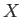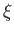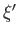Next: Measurements Up: Fundamental Concepts Previous: Eigenvalues and Eigenvectors

# Observables

We have developed a mathematical formalism that comprises three types of objects--bras, kets, and linear operators. We have already seen that kets can be used to represent the possible states of a microscopic system. However, there is a one to one correspondence between the elements of a ket space and its dual bra space, so we must conclude that bras could just as well be used to represent the states of a microscopic system. What about the dynamical variables of the system (e.g., its position, momentum, energy, spin, etc.)? How can these be represented in our formalism? Well, the only objects that we have left over are operators. We, therefore, assume that the dynamical variables of a microscopic system are represented as linear operators acting on the bras and kets that correspond to the various possible states of the system. Note that the operators have to be linear, otherwise they would, in general, spit out bras/kets pointing in different directions when fed bras/kets pointing in the same direction but differing in length. Because the lengths of bras and kets have no physical significance, it is reasonable to suppose that non-linear operators are also without physical significance.

We have seen (in Section 1.2) that if we observe the polarization state of a photon, by placing a polarizing film in its path, then the result is to cause the photon to jump into a state of polarization parallel or perpendicular to the optic axis of the film. The former state is absorbed, and the latter state is transmitted (which is how we tell them apart). In general, we cannot predict into which state a given photon will jump (except in a statistical sense). However, we do know that if the photon is initially polarized parallel to the optic axis then it will definitely be absorbed, and if it is initially polarized perpendicular to the axis then it will definitely be transmitted. We also know that, after passing though the film, a photon must be in a state of polarization perpendicular to the optic axis (otherwise it would not have been transmitted). We can make a second observation of the polarization state of such a photon by placing an identical polarizing film (with the same orientation of the optic axis) immediately behind the first film. It is clear that the photon will definitely be transmitted through the second film.

There is nothing particularly unique about the polarization states of a photon. So, more generally, we can say that if a dynamical variable of a microscopic system is measured then the system is caused to jump into one of a number of independent states (note that the perpendicular and parallel polarization states of our photon are independent). In general, each of these final states is associated with a different result of the measurement: that is, a different value of the dynamical variable. Obviously, the result of the measurement must be a real number (there are no measurement machines that output complex numbers). Finally, if an observation is made, and the system is found to be a one particular final state, with one particular value for the dynamical variable, then a second observation, made immediately after the first one, will definitely find the system in the same state, and yield the same value for the dynamical variable.

How can we represent all of these facts in our mathematical formalism? Well, by a fairly non-obvious leap of intuition, we are going to assert that a measurement of a dynamical variable corresponding to an operatorin ket space causes the system to jump into a state corresponding to one of the eigenkets of. Not surprisingly, such a state is termed an eigenstate. Furthermore, the result of the measurement is the eigenvalue associated with the eigenket into which the system jumps. The fact that the result of the measurement must be a real number implies that dynamical variables can only be represented by Hermitian operators (because only Hermitian operators are guaranteed to have real eigenvalues). The fact that the eigenkets of an Hermitian operator corresponding to different eigenvalues (i.e., different results of the measurement) are orthogonal is in accordance with our earlier requirement that the states into which the system jumps should be mutually independent. We conclude that the result of a measurement of a dynamical variable represented by an Hermitian operatormust be one of the eigenvalues of. Conversely, every eigenvalue ofis a possible result of a measurement made on the corresponding dynamical variable. This gives us the physical significance of the eigenvalues. (From now on, the distinction between a state and its representative ket, and a dynamical variable and its representative operator, will be dropped, for the sake of simplicity.)

It is reasonable to suppose that if a certain dynamical variableis measured with the system in a particular state then the states into which the system may jump on account of the measurement are such that the original state is dependent on them. This fairly innocuous statement has two very important corollaries. First, immediately after an observation whose result is a particular eigenvalue, the system is left in the associated eigenstate. However, this eigenstate is orthogonal to (i.e., independent of) any other eigenstate corresponding to a different eigenvalue. It follows that a second measurement made immediately after the first one must leave the system in an eigenstate corresponding to the eigenvalue. In other words, the second measurement is bound to give the same result as the first. Furthermore, if the system is in an eigenstate of, corresponding to an eigenvalue, then a measurement ofis bound to give the result. This follows because the system cannot jump into an eigenstate corresponding to a different eigenvalue of, because such a state is not dependent on the original state. Second, it stands to reason that a measurement ofmust always yield some result. It follows that in no matter what state the system is initially found, it must always be possible for it to jump into one of the eigenstates of. In other words, a general ket must always be dependent on the eigenkets of. This can only be the case if the eigenkets form a complete set (i.e., they span ket space). Thus, in order for an Hermitian operatorto be observable its eigenkets must form a complete set. An Hermitian operator that satisfies this condition is termed an observable. Conversely, any observable quantity must be an Hermitian operator with a complete set of eigenstates.Next: Measurements Up: Fundamental Concepts Previous: Eigenvalues and Eigenvectors
Richard Fitzpatrick 2016-01-22